# modelAccuracy

Compute R-square, RMSE, correlation, and sample mean error of predicted and observed EADs

## Syntax

``AccMeasure = modelAccuracy(eadModel,data)``
``[AccMeasure,AccData] = modelAccuracy(___,Name=Value)``

## Description

example

````AccMeasure = modelAccuracy(eadModel,data)` computes the R-square, root mean square error (RMSE), correlation, and sample mean error of observed vs. predicted exposure at default (EAD) data. `modelAccuracy` supports comparison against a reference model and also supports different correlation types. By default, `modelAccuracy` computes the metrics in the EAD scale. You can use the `ModelLevel` name-value argument to compute metrics using the underlying model's transformed scale.```

example

````[AccMeasure,AccData] = modelAccuracy(___,Name=Value)` specifies options using one or more name-value arguments in addition to the input arguments in the previous syntax.```

## Examples

collapse all

This example shows how to use `fitEADModel` to create a `Tobit` model and then use `modelAccuracy` to compute the R-Square, RMSE, correlation, and sample mean error of predicted and observed EAD.

```load EADData.mat head(EADData)```
``` UtilizationRate Age Marriage Limit Drawn EAD _______________ ___ ___________ __________ __________ __________ 0.24359 25 not married 44776 10907 44740 0.96946 44 not married 2.1405e+05 2.0751e+05 40678 0 40 married 1.6581e+05 0 1.6567e+05 0.53242 38 not married 1.7375e+05 92506 1593.5 0.2583 30 not married 26258 6782.5 54.175 0.17039 54 married 1.7357e+05 29575 576.69 0.18586 27 not married 19590 3641 998.49 0.85372 42 not married 2.0712e+05 1.7682e+05 1.6454e+05 ```
```rng('default'); NumObs = height(EADData); c = cvpartition(NumObs,'HoldOut',0.4); TrainingInd = training(c); TestInd = test(c);```

Select Model Type

Select a model type for `Tobit` or `Regression`.

`ModelType =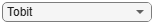"Tobit";`

Select Conversion Measure

Select a conversion measure for the EAD response values.

`ConversionMeasure =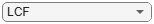"LCF";`

Create `Tobit` EAD Model

Use `fitEADModel` to create a `Tobit` model using `EADData`.

```eadModel = fitEADModel(EADData(TrainingInd,:),ModelType,PredictorVars={'UtilizationRate','Age','Marriage'}, ... ConversionMeasure=ConversionMeasure,DrawnVar="Drawn",LimitVar="Limit",ResponseVar="EAD"); disp(eadModel);```
``` Tobit with properties: CensoringSide: "both" LeftLimit: 0 RightLimit: 1 ModelID: "Tobit" Description: "" UnderlyingModel: [1x1 risk.internal.credit.TobitModel] PredictorVars: ["UtilizationRate" "Age" "Marriage"] ResponseVar: "EAD" LimitVar: "Limit" DrawnVar: "Drawn" ConversionMeasure: "lcf" ```

Display the underlying model. The underlying model's response variable is the transformation of the EAD response data. Use the `'LimitVar'` and `'DrwanVar'` name-value arguments to modify the transformation.

`disp(eadModel.UnderlyingModel);`
```Tobit regression model: EAD_lcf = max(0,min(Y*,1)) Y* ~ 1 + UtilizationRate + Age + Marriage Estimated coefficients: Estimate SE tStat pValue __________ __________ ________ __________ (Intercept) 0.22467 0.031679 7.092 1.6924e-12 UtilizationRate 0.4714 0.020683 22.791 0 Age -0.0014209 0.00077072 -1.8436 0.065356 Marriage_not married -0.010542 0.015811 -0.66676 0.50498 (Sigma) 0.3618 0.0049791 72.665 0 Number of observations: 2627 Number of left-censored observations: 0 Number of uncensored observations: 2626 Number of right-censored observations: 1 Log-likelihood: -1057.9 ```

EAD prediction operates on the underlying compact statistical model and then transforms the predicted values back to the EAD scale. You can specify the `predict` function with different options for the `'ModelLevel'` name-value argument.

```predictedEAD = predict(eadModel,EADData(TestInd,:),ModelLevel="ead"); predictedConversion = predict(eadModel,EADData(TestInd,:),ModelLevel="ConversionMeasure");```

For model validation, use `modelDiscrimination`, `modelDiscriminationPlot`, `modelAccuracy`, and `modelAccuracyPlot`.

Use `modelDiscrimination` and then `modelDiscriminationPlot` to plot the ROC curve.

```ModelLevel ="ead"; [DiscMeasure1,DiscData1] = modelDiscrimination(eadModel,EADData(TestInd,:),ModelLevel=ModelLevel); modelDiscriminationPlot(eadModel,EADData(TestInd, :),ModelLevel=ModelLevel,SegmentBy="Marriage");```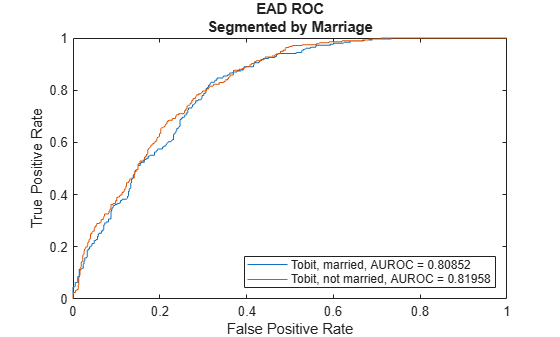Use `modelAccuracy` and then `modelAccuracyPlot` to show a scatter plot of the predictions.

```YData =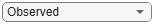"Observed"; [AccMeasure1,AccData1] = modelAccuracy(eadModel,EADData(TestInd,:),ModelLevel=ModelLevel)```
```AccMeasure1=1×4 table RSquared RMSE Correlation SampleMeanError ________ _____ ___________ _______________ Tobit 0.3919 42494 0.62602 -1240.7 ```
```AccData1=1751×3 table Observed Predicted_Tobit Residuals_Tobit __________ _______________ _______________ 44740 14893 29847 54.175 8730.2 -8676 987.39 13244 -12257 9606.4 7367.5 2238.9 83.809 27501 -27417 73538 45726 27812 96.949 5522.5 -5425.5 873.21 4426.3 -3553.1 328.35 5952.4 -5624.1 55237 28040 27198 30359 19047 11312 39211 28368 10843 2.0885e+05 1.0539e+05 1.0346e+05 1921.7 19939 -18017 15230 5427.4 9802.5 20063 9359.6 10703 ⋮ ```
`modelAccuracyPlot(eadModel,EADData(TestInd,:),ModelLevel=ModelLevel,YData=YData);`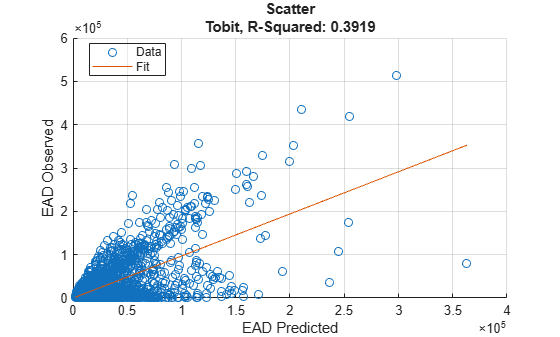This example shows how to use `fitEADModel` to create a `Beta` model and then use `modelAccuracy` to compute the R-Square, RMSE, correlation, and sample mean error of predicted and observed EAD.

```load EADData.mat head(EADData)```
``` UtilizationRate Age Marriage Limit Drawn EAD _______________ ___ ___________ __________ __________ __________ 0.24359 25 not married 44776 10907 44740 0.96946 44 not married 2.1405e+05 2.0751e+05 40678 0 40 married 1.6581e+05 0 1.6567e+05 0.53242 38 not married 1.7375e+05 92506 1593.5 0.2583 30 not married 26258 6782.5 54.175 0.17039 54 married 1.7357e+05 29575 576.69 0.18586 27 not married 19590 3641 998.49 0.85372 42 not married 2.0712e+05 1.7682e+05 1.6454e+05 ```
```rng('default'); NumObs = height(EADData); c = cvpartition(NumObs,'HoldOut',0.4); TrainingInd = training(c); TestInd = test(c);```

Select Model Type

Select a model type for `Beta`.

`ModelType =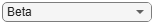"Beta";`

Select Conversion Measure

Select a conversion measure for the EAD response values.

`ConversionMeasure ="LCF";`

Create `Beta` EAD Model

Use `fitEADModel` to create a `Beta` model using the `TrainingInd` data.

```eadModel = fitEADModel(EADData(TrainingInd,:),ModelType,PredictorVars={'UtilizationRate','Age','Marriage'}, ... ConversionMeasure=ConversionMeasure,DrawnVar="Drawn",LimitVar="Limit",ResponseVar="EAD"); disp(eadModel);```
``` Beta with properties: BoundaryTolerance: 1.0000e-07 ModelID: "Beta" Description: "" UnderlyingModel: [1x1 risk.internal.credit.BetaModel] PredictorVars: ["UtilizationRate" "Age" "Marriage"] ResponseVar: "EAD" LimitVar: "Limit" DrawnVar: "Drawn" ConversionMeasure: "lcf" ```

Display the underlying model. The underlying model's response variable is the transformation of the EAD response data. Use the `'LimitVar'` and `'DrwanVar'` name-value arguments to modify the transformation.

`disp(eadModel.UnderlyingModel);`
```Beta regression model: logit(EAD_lcf) ~ 1_mu + UtilizationRate_mu + Age_mu + Marriage_mu log(EAD_lcf) ~ 1_phi + UtilizationRate_phi + Age_phi + Marriage_phi Estimated coefficients: Estimate SE tStat pValue __________ _________ ________ __________ (Intercept)_mu -0.65566 0.11484 -5.7093 1.2616e-08 UtilizationRate_mu 1.7014 0.078094 21.787 0 Age_mu -0.0055901 0.0027603 -2.0252 0.042949 Marriage_not married_mu -0.012577 0.052098 -0.24141 0.80926 (Intercept)_phi -0.50131 0.094625 -5.2979 1.2686e-07 UtilizationRate_phi 0.39731 0.066707 5.956 2.9303e-09 Age_phi -0.001167 0.0023161 -0.50387 0.6144 Marriage_not married_phi -0.013275 0.042627 -0.31143 0.7555 Number of observations: 2627 Log-likelihood: -3140.21 ```

EAD prediction operates on the underlying compact statistical model and then transforms the predicted values back to the EAD scale. You can specify the `predict` function with different options for the `'ModelLevel'` name-value argument.

```predictedEAD = predict(eadModel,EADData(TestInd,:),ModelLevel="ead"); predictedConversion = predict(eadModel,EADData(TestInd,:),ModelLevel="ConversionMeasure");```

For model validation, use `modelDiscrimination`, `modelDiscriminationPlot`, `modelAccuracy`, and `modelAccuracyPlot`.

Use `modelDiscrimination` and then `modelDiscriminationPlot` to plot the ROC curve.

```ModelLevel =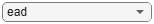"ead"; [DiscMeasure1,DiscData1] = modelDiscrimination(eadModel,EADData(TestInd,:),ModelLevel=ModelLevel); modelDiscriminationPlot(eadModel,EADData(TestInd, :),ModelLevel=ModelLevel,SegmentBy="Marriage");```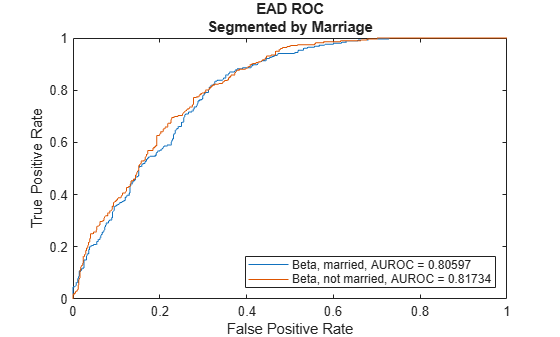Use `modelAccuracy` and then `modelAccuracyPlot` to show a scatter plot of the predictions.

```YData ="Observed"; [AccMeasure1,AccData1] = modelAccuracy(eadModel,EADData(TestInd,:),ModelLevel=ModelLevel)```
```AccMeasure1=1×4 table RSquared RMSE Correlation SampleMeanError ________ _____ ___________ _______________ Beta 0.38655 43817 0.62173 -7393.4 ```
```AccData1=1751×3 table Observed Predicted_Beta Residuals_Beta __________ ______________ ______________ 44740 18039 26701 54.175 10560 -10506 987.39 15551 -14564 9606.4 8407.7 1198.8 83.809 33318 -33234 73538 52120 21418 96.949 6598.1 -6501.2 873.21 5471.1 -4597.9 328.35 7335 -7006.6 55237 32580 22658 30359 21563 8796.4 39211 33177 6033.6 2.0885e+05 1.2586e+05 82987 1921.7 23319 -21397 15230 6565.9 8664 20063 11075 8987.5 ⋮ ```
`modelAccuracyPlot(eadModel,EADData(TestInd,:),ModelLevel=ModelLevel,YData=YData);`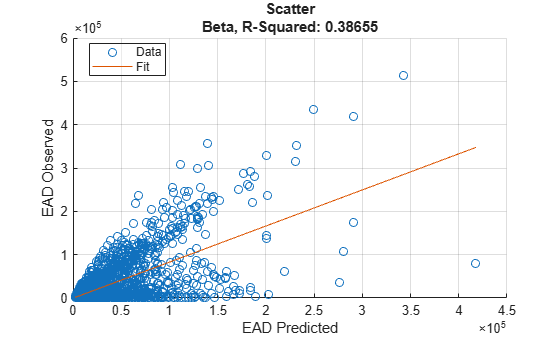## Input Arguments

collapse all

Loss given default model, specified as a previously created `Regression`, `Tobit`, or `Beta` object using `fitEADModel`.

Data Types: `object`

Data, specified as a `NumRows`-by-`NumCols` table with predictor and response values. The variable names and data types must be consistent with the underlying model.

Data Types: `table`

### Name-Value Arguments

Specify optional pairs of arguments as `Name1=Value1,...,NameN=ValueN`, where `Name` is the argument name and `Value` is the corresponding value. Name-value arguments must appear after other arguments, but the order of the pairs does not matter.

Example: ```[AccMeasure,AccData] = modelAccuracy(eadModel,data(TestInd,:),DataID='Testing',CorrelationType='spearman')```

Correlation type, specified as `CorrelationType` and a character vector or string.

Data Types: `char` | `string`

Data set identifier, specified as `DataID` and a character vector or string. The `DataID` is included in the output for reporting purposes.

Data Types: `char` | `string`

Model level, specified as `ModelLevel` and a character vector or string.

Note

`Regression` models support all three model levels, but a `Tobit` or `Beta` model supports model levels only for `"ead"` and `"conversionMeasure"`.

Data Types: `char` | `string`

EAD values predicted for `data` by the reference model, specified as `ReferenceEAD` and a `NumRows`-by-`1` numeric vector. The `modelAccuracy` output information is reported for both the `eadModel` object and the reference model.

Data Types: `double`

Identifier for the reference model, specified as `ReferenceID` and a character vector or string. `ReferenceID` is used in the `modelAccuracy` output for reporting purposes.

Data Types: `char` | `string`

## Output Arguments

collapse all

Accuracy measure, returned as a table with columns `'RSquared'`, `'RMSE'`, `'Correlation'`, and `'SampleMeanError'`. `AccMeasure` has one row if only the `eadModel` accuracy is measured and it has two rows if reference model information is given. The row names of `AccMeasure` report the model ID and data ID (if provided).

Accuracy data, returned as a table with observed EAD values, predicted EAD values, and residuals (observed minus predicted). Additional columns for predicted and residual values are included for the reference model, if provided. The `ModelID` and `ReferenceID` labels are appended in the column names.

collapse all

### Model Accuracy

Model accuracy measures the accuracy of the predicted probability of EAD values using different metrics.

• R-squared — To compute the R-squared metric, `modelAccuracy` fits a linear regression of the observed EAD values against the predicted EAD values:

`$EA{D}_{obs}=a+b\ast EA{D}_{pred}+\epsilon$`

The R-square of this regression is reported. For more information, see Coefficient of Determination (R-Squared).

• RMSE — To compute the root mean square error (RMSE), `modelAccuracy` uses the following formula where N is the number of observations:

`$RMSE=\sqrt{\frac{1}{N}{\sum }_{i=1}^{N}\left(EA{D}_{i}^{obs}-EA{D}_{i}^{pred}{\right)}^{2}}$`

• Correlation — This metric is the correlation between the observed and predicted EAD:

`$corr\left(EA{D}_{obs},EA{D}_{pred}\right)$`

For more information and details about the different correlation types, see `corr`.

• Sample mean error — This metric is the difference between the mean observed EAD and the mean predicted EAD or, equivalently, the mean of the residuals:

`$SampleMeanError=\frac{1}{N}{\sum }_{i=1}^{N}\left(EA{D}_{i}^{obs}-EA{D}_{i}^{pred}\right)$`

 Baesens, Bart, Daniel Roesch, and Harald Scheule. Credit Risk Analytics: Measurement Techniques, Applications, and Examples in SAS. Wiley, 2016.

 Bellini, Tiziano. IFRS 9 and CECL Credit Risk Modelling and Validation: A Practical Guide with Examples Worked in R and SAS. San Diego, CA: Elsevier, 2019.

 Brown, Iain. Developing Credit Risk Models Using SAS Enterprise Miner and SAS/STAT: Theory and Applications. SAS Institute, 2014.

 Roesch, Daniel and Harald Scheule. Deep Credit Risk. Independently published, 2020.